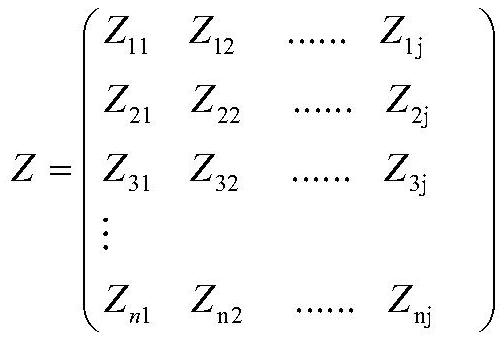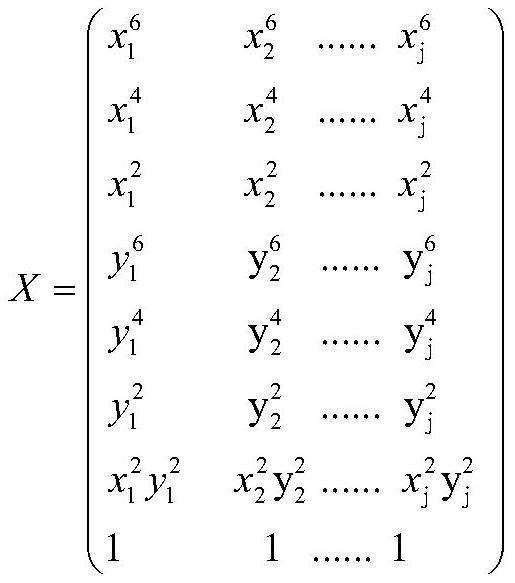# Measuring system and measuring method

## A measurement system and measurement method technology, applied in special data processing applications, complex mathematical operations, design optimization/simulation, etc., can solve problems such as low detection accuracy

Pending Publication Date: 2022-03-01

2 Cites 0 Cited by

## AI-Extracted Technical Summary

### Problems solved by technology

 The purpose of the present invention is to solve the problem of low detection accuracy of exist...
View more

### Method used

Step 3, position correction: according to the mathematical model between the impedance obtained in step 1 and the cell position and the coordinate position of the cell recorded in ...
View more

## Abstract

The invention relates to a measurement system and a measurement method, and the technical key points are as follows: firstly, obtaining a characteristic relation between cell position change and impedance through a simulation mode, and establishing a mathematical model between the characteristic relation and the impedance; secondly, correcting the impedance data according to the mathematical model so as to eliminate the influence of the position on the impedance and improve the measurement precision; and finally, extracting the electrical characteristics of the biological embryo through an impedance spectrum equivalent circuit automatic fitting algorithm. The measuring system and the measuring method provided by the invention are of great significance to research on drug response and cell development.

Application Domain

Design optimisation/simulationMaterial impedance +1

Technology Topic

PhysicsCellular development +9

## Image

•••## Examples

• Experimental program(4)

### Example Embodiment

 [Example 1: Overall Framework Design of Accurate Measurement Method]
 The precise measurement method of this application and a measurement system are combined below figure 1 Further description of the invention.
 Precision measurement method of electrical characteristics in embryonic development, including the following steps:
 Step 1, the characteristic relationship between cell position and impedance: obtains a value of cell position and impedance by numerical simulation;
 Use polynomial to fit the mathematical model between the impedance and cell line;
 Step 2, measure the impedance of the cells in the sensor, and observe the coordinate position of the cells by electron microscopy;
 Step 3, position correction: According to the mathematical model between the impedance and the cell line, the math model between the cells and the coordinate position of the cells recorded, the impedance of the step II is corrected, thereby avoiding the effect of position factors on impedance, implementation High precision measurement.
 Step 4, according to the three-step data, the self-proportion of the electrical characteristic parameters is achieved by the biological impedance spectrum equivalent circuit.
 In response to the above measurement method, an embodiment further proposes a measurement system, which is constituted and action.
 Table 1


 For step four, the electrical properties are extracted by the biological resistance spectrum equivalent circuit self-propelled algorithm; the automatic fitting algorithm is a mixed algorithm in which the genetic expression algorithm is combined with genetic algorithm, with genetic expression algorithm to achieve biological The construction of the impedance spectrum equivalent circuit, the genetic algorithm is based on the constructed equivalent circuit model to achieve the fitting of biological impedance spectroscopy and the calculation of electrical parameters.

### Example Embodiment

 [Example 2: Specific work in numerical simulation system]
 The specific work of the numerical simulation system, the corresponding is the step of step.
 For steps, through numerical simulation, acquire the position and impedance mathematical model of the cell in the sensor, including:
 S1-1, grid division for the simulation area;
 S1-2, randomly select any of the positions as the center coordinate position of the embryo, its coordinate expression is (X c Y c ), Frequency f is any frequency f b And measured the obtained impedance value expression as Z bc;
 Central coordinates Select the J group and the frequency selects N group;
 Then, the impedance value matrix z is recorded:

 Record position matrix x:

 S1-3, solve matrix A:

 The above matrix is ​​expressed in the formula: z = a · x
 It can be seen: a = zx -1 (即 can solve the various values ​​in the matrix A)
 in,

 S1-4, establishing an impedance and cell position to fit the corresponding mathematical model:


### Example Embodiment

 [Example 3: Specific work in position correction system]
 For the method of the embodiment, one of the problems is "how to perform position correction", which is specifically in the actual measurement process, which causes the embryo to be in different positions of the sensor; When the embryo is in different locations of the sensor, the impedance of the measurement is different, and this is difficult to evaluate the effect.
 That is, each measurement, the actual coordinate position of the embryo in the sensor (X p Y p ) It is inevitably different, and there is a need to convert different coordinate positions to the same coordinate position (reference position (X) r Y r )) The comparative analysis is carried out, in turn to avoid the effect of the positional factor on the impedance to obtain high-precision measurement results.
 For this issue, processes the following:
 The steps of their correction process are as follows:
 Step Second to record the actual location of the embryo in the sensor (X p Y p ), The frequency of its record is f g Impedance is Z g;
 Translate into the reference position (X r Y r The impedance is Z ' g :


## PUM## Description & Claims & Application Information

We can also present the details of the Description, Claims and Application information to help users get a comprehensive understanding of the technical details of the patent, such as background art, summary of invention, brief description of drawings, description of embodiments, and other original content. On the other hand, users can also determine the specific scope of protection of the technology through the list of claims; as well as understand the changes in the life cycle of the technology with the presentation of the patent timeline. Login to view more.
Who we serve
• R&D Engineer
• R&D Manager
• IP Professional
Why Eureka